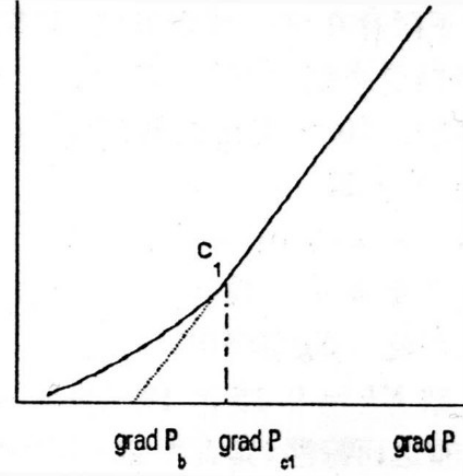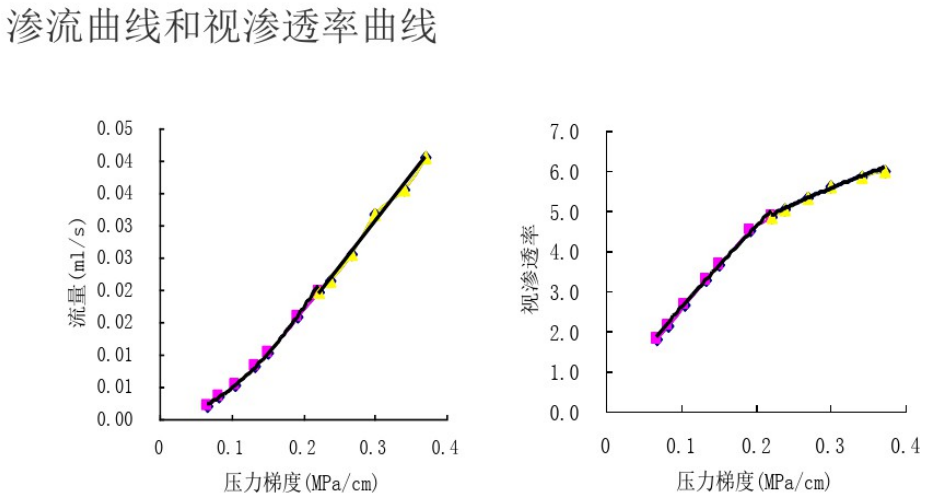# 渗透率

## 渗透率的分类

### 相对渗透率

$$K_{ro} = K_o / k$$
$$K_{rg} = K_g / k$$
$$K_{rw} = K_w / k$$

（1）砂岩颗粒的形状和大小；
（2）层理；
（3）胶结作用；
（4）破碎和溶解作用。

## 单位

$Q({cm^3} / s) = \frac{ K(D) × A({cm^2}) }{ μ(mPa·s) } \frac{ \Delta p(atm)}{L (cm) }$

$1D = 0.986923 × 10^{-12} {m}^2 = 0.986923 × 10^{-8} {cm}^2 = 0.986923 {μm}^2$

# 达西定律

1856年，发过水力工程师Henry Darcy在研究净化用的砂滤器中水的流动时提出的流体渗流方程，已成为石油工程师计算渗透率标准公式之一。

## 适用条件

• 渗流流体为单相牛顿流体，服从牛顿内摩擦定律，应力与应变呈线性关系；
• 多孔介质性质稳定。渗流过程中孔隙结构保持不变，反应多孔介质渗流能力的渗透率值为一常数；
• 多孔介质性质和流体性质分别为渗流方程中的独立参数。多孔介质和渗流流体之间的相互作用对流体流动的影响很小，可以忽略；
• 多孔介质中流体以层状态流动；
• 达西定律适用于黏性流，即流体的流速足够低，且与压力梯度城正比。
• 流体不予多孔介质发生化学反应。

## 简单微分形式

$$v = \frac{q}{A_c} = - \frac{K}{μ} \frac{dp}{dL}$$

# 绝对渗透率实验

## 气体法绝对渗透率

$K(D) = \frac{Q_{av} μ L}{A (p_2 - p_1)}$

# Forchheimer惯性阻力

达西定律具有局限性，仅限于低速体积流量。

# 相对渗透率实验

$$K_{rg} = \frac{k_{eg}}{K}$$

$$K_{rw} = \frac{k_{ew}}{K}$$

$$K_{ro} = \frac{k_{eo}}{K}$$

## 稳态法

### 实验方法

$$K_{ew} = \frac{Q_w μ_w L}{A \Delta p_0} = \mathcal{f} (S_w)$$

$$K_{eo} = \frac{Q_o μ_o L}{A \Delta p_0} = \mathcal{f} (S_w)$$

$$M = (D_M) + S_w V_p \rho + (1-S_w)V_pp_0$$

$$S_w = \frac{M - D_M - V_p \rho_o}{V_p(\rho_w - \rho_o)}$$

## 相对渗透率测量设备及步骤

（1）使岩心100%饱水，用油驱水至不再出水为止（$$S_{wi}$$）。用离心机分离驱替出的油水，计量被油驱出的水量，计算初始含水饱和度。
（2）静置岩心12小时，使其达到毛管平衡。
（3）对于恒速注入，调节定量泵，使驱替液以恒定流速进入岩心。对于恒压注入，调整气驱柱塞泵以恒定压力输送驱替液。
（4）开启驱替泵，并计时。
（5）若使用水和原油驱替系统，用带刻度的离心集液管计量出口的液体并计时；若使用气液驱替系统，用气体流量计计量出口的气体，用带刻度的量筒计量出口的液体并计时。
（6）当出口躯体液中不再含油时或达到预定注入水体积后，停止实验（本实验为3倍孔隙体积）。
（7）如果使用原油，用离心机分离油和水，记录每一段时间驱除的油水量及时间。
（8）计算累计油水量，以注入孔隙体积倍数为横坐标绘制累积出油曲线。
（9）对没一时段计算油水的流率、相对渗透率和平均含水饱和度$$S_{w(av)}$$

$$S_{w(av)} = S_{wi} + \frac{V_{oil(produced)}}{V_p}$$

# 低渗非达西流

## 特征曲线

### 非线性段

$$Q=a_1 (grad P)^n$$

### 拟启动压力梯度• 非牛顿流体渗流
• 非层流流态渗流
• 变形多孔介质渗流
• 非等温渗流
• 低渗多孔介质渗流

## 非达西流渗流条件

• 低渗多孔介质孔隙喉道细小，流体与孔隙介质之间的作用力对流体的影响已不能忽略。
• 细小孔隙喉道，油气是细小喉道存在明显的启动压力梯度。
• 使渗流规律不具备达西渗流所使用的渗流条件，呈现低渗非达西渗流特征。

## 低渗非达西渗流机理

### 启动压力梯度

• 固液界面存在分子作用力，成吸附滞留层。
• 吸附滞留层的厚度约为0.1μm。
• 低渗多孔介质孔隙吼道更细小，孔径和吸附滞留层厚度在同一数量级，甚至更小。
• 细小孔隙中，吸附滞留层对流体流动的影响不可忽略，存在启动压力梯度。

### 流动孔隙数

• 储层中的孔隙系统是由无数孔径大小不等的孔隙组成。
• 孔径越小，启动压力越大。
• 在非线性段，随压力梯度的增大，参与流动的孔隙数增多。
• 达到临界压力梯度以后，流动孔隙数成为定值。

### 附加渗流阻力

• 拟启动压力梯度反应附加渗流阻力。
• 在非线性段，岁流动孔隙数增多附加渗流阻力增大。
• 在拟线性段，附加渗流阻力位定值。
• 不管是非线性段还是拟线性段，附加渗流阻力的影响都存在。

## 低渗非达西渗流的综合判据

### 渗流状态的影响因素

1. 从中高达西渗流到低渗非达西渗流是个渐变过程。
2. 两种渗流状态的判别标准并非是渗透率的单值函数。
3. 它取决与多孔介质孔隙结构参数和流体性质参数：
4. 1：孔隙结构参数：孔隙半径、喉道半径、孔吼比、配位数、孔隙迂回度、孔隙分布密度等。
5. 2：流体性质参数：流体黏度和密度。

### 低渗非达西渗流的判别依据

#### 低渗非达西渗流特征判别依据

• 拟启动压力梯度。拟启动压力梯度为0，即达西线性渗透；拟启动压力梯度>0，即为低渗非达西渗流。

### 低渗非达西渗流的判别式

$${\lambda}_N = \frac{μ^2}{Kρ} \frac{R}{r}$$
$${\lambda}_N$$ 的单位为Pa，称为压力数

2<压力数<5，视为过渡区。

## 低渗非达西渗流的视渗透率

### 视渗透率

$$K_s = \frac{Q μ}{A gradP}$$

### 视渗透率曲线### 视渗透率变化特征

• 视渗透率远比气测渗透率低。
• 视渗透率是变量，与压力梯度直接有关。压力梯度月底，视渗透率越低。
• 大于临界压力梯度的情况下，视渗透率明显较高。
• 小于临界压力梯度时，视渗透率明显较低。